# Solving Literal Equations Use inverse operations to solve

• Slides: 10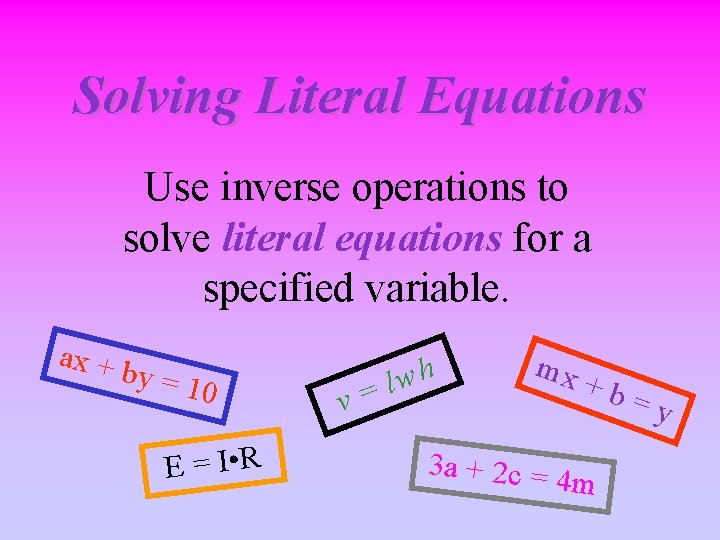Solving Literal Equations Use inverse operations to solve literal equations for a specified variable. ax + b y = 10 E = I • R v= lwh mx +b 3 a + 2 c = 4 m =yReview Inverse Operations 5 x – 2 = 8 +2 +2 5 x = 10 5 5 x=2 To solve for x, we need to get rid of the 5 and the – 2. Which one first? HOW? Add 2 to both sides. Next, get rid of the 5. HOW? Divide both sides by 5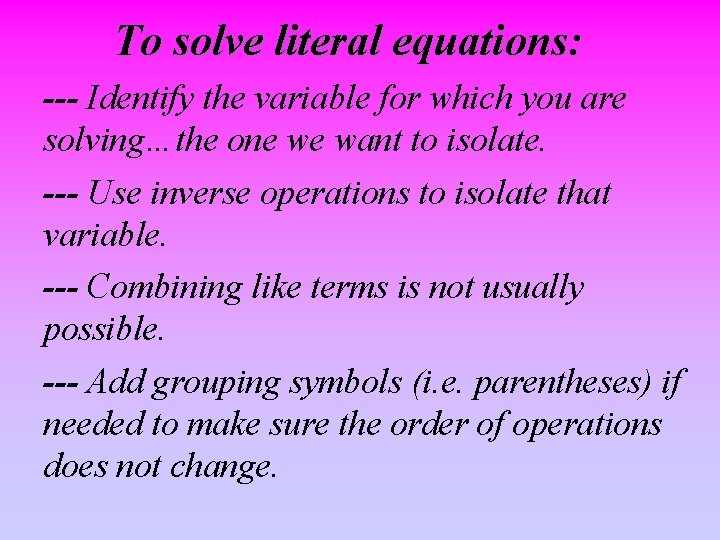To solve literal equations: --- Identify the variable for which you are solving…the one we want to isolate. --- Use inverse operations to isolate that variable. --- Combining like terms is not usually possible. --- Add grouping symbols (i. e. parentheses) if needed to make sure the order of operations does not change.Solve m + a = p for m. m+a=p –a –a Identify the variable for which you need to solve. We want to isolate the variable, m. We need to get rid of the + a. Subtract a from both sides. m=p–a Since p and a are not like terms, we cannot combine them.Solve V = l·w·h for w. V = l·w·h lh lh V =w l • h V w= l • h Identify the variable for which you need to solve. We want to isolate the variable, w. Since w is multiplied times l, we need to get rid of it. How? Divide both sides by l. Since w is also multiplied times h, we need to get rid of it. How? Divide both sides by h. Write the equation with the isolated variable first.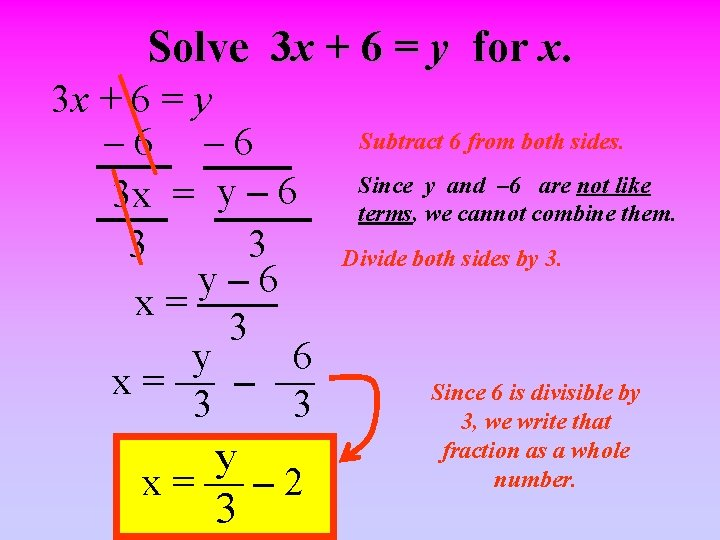Solve 3 x + 6 = y for x. 3 x + 6 = y – 6 3 x = y – 6 3 3 y– 6 x = —— 3 y 6 x=— – — 3 3 y x=—– 2 3 Subtract 6 from both sides. Since y and – 6 are not like terms, we cannot combine them. Divide both sides by 3. Since 6 is divisible by 3, we write that fraction as a whole number.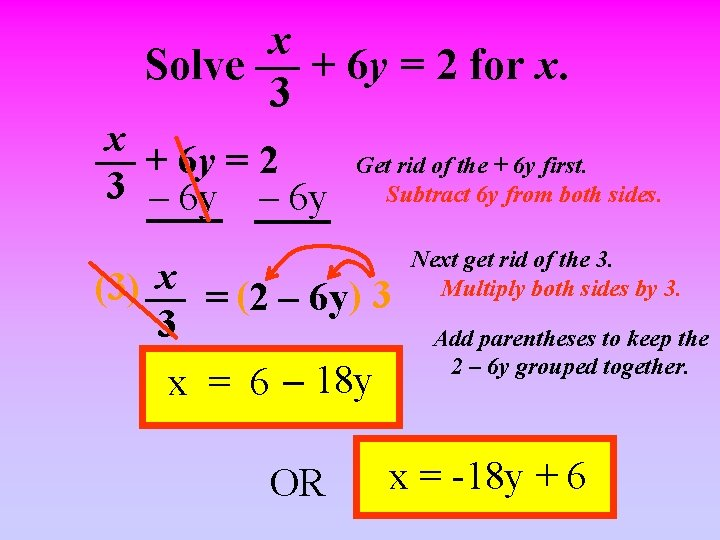x Solve — + 6 y = 2 for x. 3 x — + 6 y = 2 3 – 6 y Get rid of the + 6 y first. Subtract 6 y from both sides. x ( (3) — = 2 – 6 y) 3 3 x = 6 – 18 y OR Next get rid of the 3. Multiply both sides by 3. Add parentheses to keep the 2 – 6 y grouped together. x = -18 y + 6Solve ax + b = y for x ax + b = y –b –b Subtract b from both sides. ax = y – b Since y and b are not like terms, a a we cannot combine them. y–b Divide both sides of the x= a equation by a. OR y x= a b a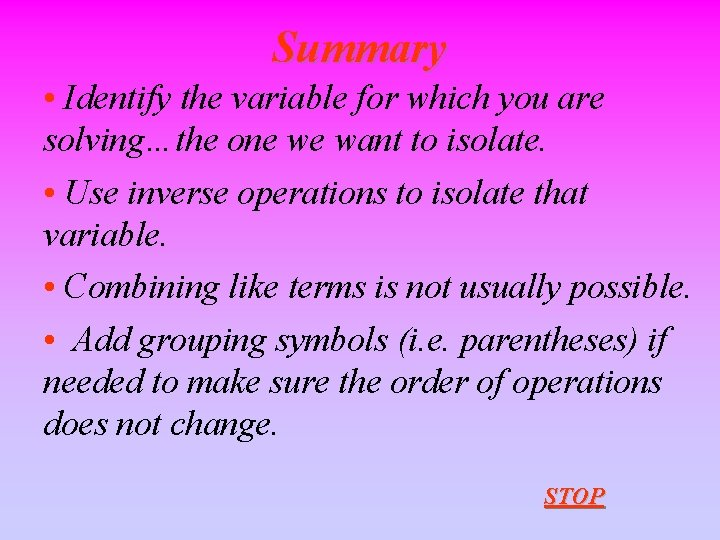Summary • Identify the variable for which you are solving…the one we want to isolate. • Use inverse operations to isolate that variable. • Combining like terms is not usually possible. • Add grouping symbols (i. e. parentheses) if needed to make sure the order of operations does not change. STOP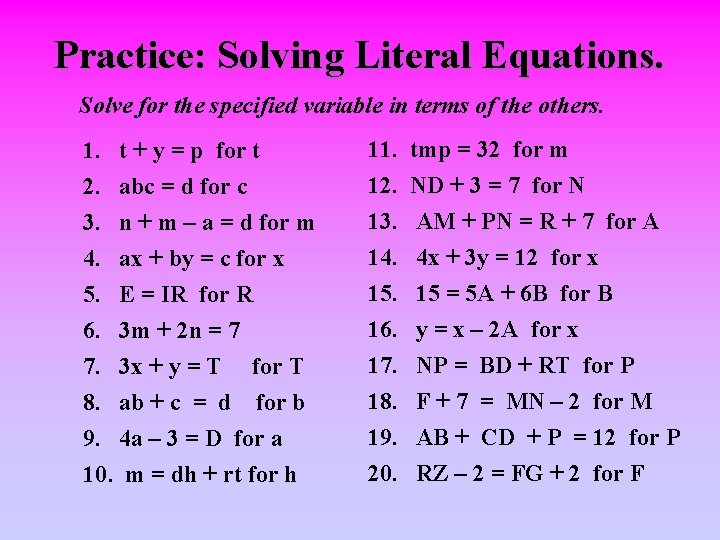Practice: Solving Literal Equations. Solve for the specified variable in terms of the others. 1. t + y = p for t 2. abc = d for c 3. n + m – a = d for m 4. ax + by = c for x 5. E = IR for R 6. 3 m + 2 n = 7 7. 3 x + y = T for T 8. ab + c = d for b 9. 4 a – 3 = D for a 10. m = dh + rt for h 11. 12. 13. 14. 15. 16. 17. 18. 19. 20. tmp = 32 for m ND + 3 = 7 for N AM + PN = R + 7 for A 4 x + 3 y = 12 for x 15 = 5 A + 6 B for B y = x – 2 A for x NP = BD + RT for P F + 7 = MN – 2 for M AB + CD + P = 12 for P RZ – 2 = FG + 2 for F